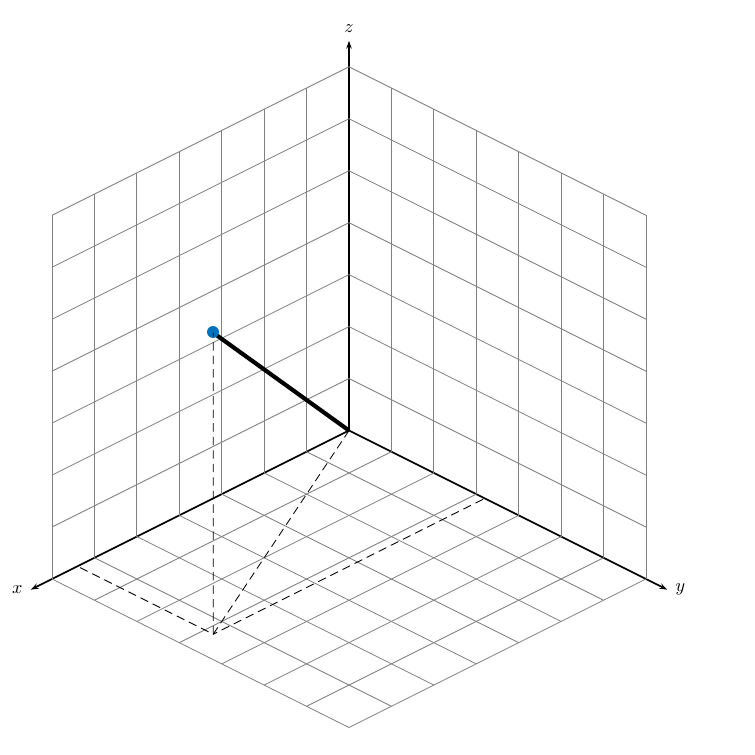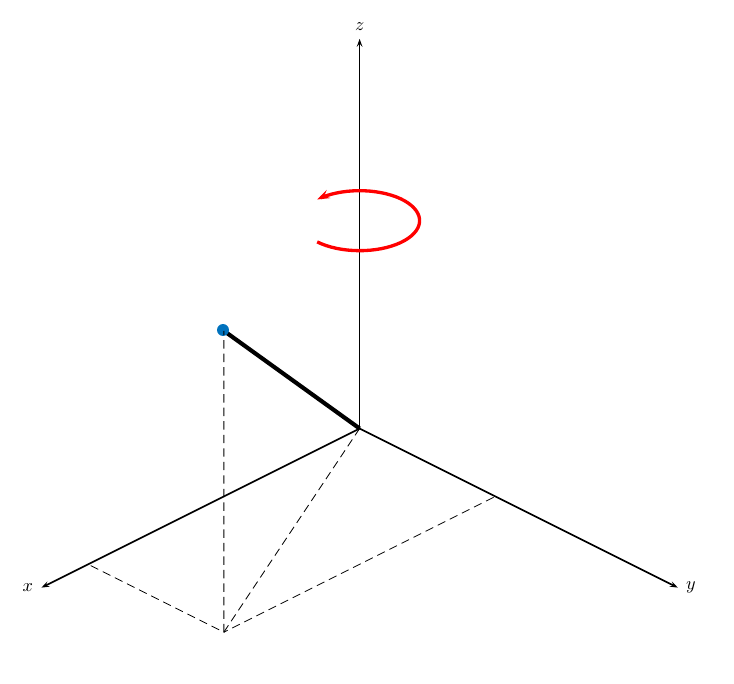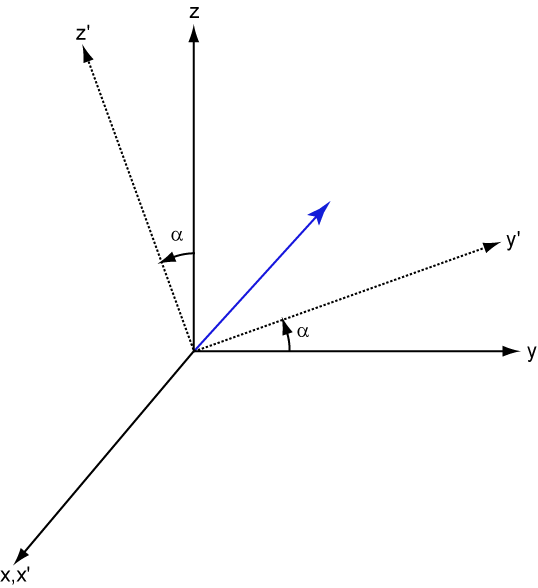Main Content

## Rectangular Coordinates

### Definitions of Coordinates

Construct a rectangular, or Cartesian, coordinate system for three-dimensional space by specifying three mutually orthogonal coordinate axes. The following figure shows one possible specification of the coordinate axes.Rectangular coordinates specify a position in space in a given coordinate system as an ordered 3-tuple of real numbers, (x,y,z), with respect to the origin (0,0,0). Considerations for choosing the origin are discussed in Global and Local Coordinate Systems.

You can view the 3-tuple as a point in space, or equivalently as a vector in three-dimensional Euclidean space. Viewed as a vector space, the coordinate axes are basis vectors and the vector gives the direction to a point in space from the origin. Every vector in space is uniquely determined by a linear combination of the basis vectors. The most common set of basis vectors for three-dimensional Euclidean space are the standard unit basis vectors:

`$\left\{\left[1\text{\hspace{0.17em}}\text{ }\text{ }0\text{\hspace{0.17em}}0\right],\left[0\text{\hspace{0.17em}}1\text{\hspace{0.17em}}0\right],\left[0\text{\hspace{0.17em}}0\text{\hspace{0.17em}}1\right]\right\}$`

### Notation for Vectors and Points

In Phased Array System Toolbox™ software, you specify both coordinate axes and points as column vectors.

Note

In this software, all coordinate vectors are column vectors. For convenience, the documentation represents column vectors in the format [x y z] without transpose notation.

Both the vector notation [x y z] and point notation (x,y,z) are used interchangeably. The interpretation of the column vector as a vector or point depends on the context. If the column vector specifies the axes of a coordinate system or direction, it is a vector. If the column vector specifies coordinates, it is a point.

### Orthogonal Basis and Euclidean Norm

Any three linearly independent vectors define a basis for three-dimensional space. However, this software assumes that the basis vectors you use are orthogonal.

The standard distance measure in space is the l2 norm, or Euclidean norm. The Euclidean norm of a vector [x y z] is defined by:

`$\sqrt{{x}^{2}+{y}^{2}+{z}^{2}}$`

The Euclidean norm gives the length of the vector measured from the origin as the hypotenuse of a right triangle. The distance between two vectors [x0 y0 z0] and [x1 y1 z1] is:

`$\sqrt{{\left({x}_{0}-{x}_{1}\right)}^{2}+{\left({y}_{0}-{y}_{1}\right)}^{2}+{\left({z}_{0}-{z}_{1}\right)}^{2}}$`

### Orientation of Coordinate Axes

Given an orthonormal set of basis vectors representing the coordinate axes, there are multiple ways to orient the axes. The following figure illustrates one such orientation, called a right-handed coordinate system. The arrows on the coordinate axes indicate the positive directions.If you take your right hand and point it along the positive x-axis with your palm facing the positive y-axis and extend your thumb, your thumb indicates the positive direction of the z-axis.

### Rotations and Rotation Matrices

In transforming vectors in three-dimensional space, rotation matrices are often encountered. Rotation matrices are used in two senses: they can be used to rotate a vector into a new position or they can be used to rotate a coordinate basis (or coordinate system) into a new one. In this case, the vector is left alone but its components in the new basis will be different from those in the original basis. In Euclidean space, there are three basic rotations: one each around the x, y and z axes. Each rotation is specified by an angle of rotation. The rotation angle is defined to be positive for a rotation that is counterclockwise when viewed by an observer looking along the rotation axis towards the origin. Any arbitrary rotation can be composed of a combination of these three (Euler’s rotation theorem). For example, you can rotate a vector in any direction using a sequence of three rotations: ${v}^{\prime }=Av={R}_{z}\left(\gamma \right){R}_{y}\left(\beta \right){R}_{x}\left(\alpha \right)v$.

The rotation matrices that rotate a vector around the x, y, and z-axes are given by:

• Counterclockwise rotation around x-axis

`${R}_{x}\left(\alpha \right)=\left[\begin{array}{ccc}1& 0& 0\\ 0& \mathrm{cos}\alpha & -\mathrm{sin}\alpha \\ 0& \mathrm{sin}\alpha & \mathrm{cos}\alpha \end{array}\right]$`

• Counterclockwise rotation around y-axis

`${R}_{y}\left(\beta \right)=\left[\begin{array}{ccc}\mathrm{cos}\beta & 0& \mathrm{sin}\beta \\ 0& 1& 0\\ -\mathrm{sin}\beta & 0& \mathrm{cos}\beta \end{array}\right]$`

• Counterclockwise rotation around z-axis

`${R}_{z}\left(\gamma \right)=\left[\begin{array}{ccc}\mathrm{cos}\gamma & -\mathrm{sin}\gamma & 0\\ \mathrm{sin}\gamma & \mathrm{cos}\gamma & 0\\ 0& 0& 1\end{array}\right]$`

The following three figures show what positive rotations look like for each rotation axis:For any rotation, there is an inverse rotation satisfying ${A}^{-1}A=1$. For example, the inverse of the x-axis rotation matrix is obtained by changing the sign of the angle:

`${R}_{x}^{-1}\left(\alpha \right)={R}_{x}\left(-\alpha \right)=\left[\begin{array}{ccc}1& 0& 0\\ 0& \mathrm{cos}\alpha & \mathrm{sin}\alpha \\ 0& -\mathrm{sin}\alpha & \mathrm{cos}\alpha \end{array}\right]={R}_{x}^{\prime }\left(\alpha \right)$`

This example illustrates a basic property: the inverse rotation matrix is the transpose of the original. Rotation matrices satisfy A’A = 1, and consequently det(A) = 1. Under rotations, vector lengths are preserved as well as the angles between vectors.

We can think of rotations in another way. Consider the original set of basis vectors, $i,j,k$, and rotate them all using the rotation matrix A. This produces a new set of basis vectors ${i}^{\prime },j{,}^{\prime }{k}^{\prime }$ related to the original by:

`$\begin{array}{ll}{i}^{\prime }\hfill & =Ai\hfill \\ {j}^{\prime }\hfill & =Aj\hfill \\ {k}^{\prime }\hfill & =Ak\hfill \end{array}$`

Using the transpose, you can write the new basis vectors as a linear combinations of the old basis vectors:

`$\left[\begin{array}{c}{i}^{\prime }\\ {j}^{\prime }\\ {k}^{\prime }\end{array}\right]={A}^{\prime }\left[\begin{array}{c}i\\ j\\ k\end{array}\right]$`

Now any vector can be written as a linear combination of either set of basis vectors:

`$v={v}_{x}i+{v}_{y}j+{v}_{z}k={{v}^{\prime }}_{x}{i}^{\prime }+{{v}^{\prime }}_{y}{j}^{\prime }+{{v}^{\prime }}_{z}{k}^{\prime }$`

Using algebraic manipulation, you can derive the transformation of components for a fixed vector when the basis (or coordinate system) rotates. This transformation uses the transpose of the rotation matrix.

`$\left[\begin{array}{c}{{v}^{\prime }}_{x}\\ {{v}^{\prime }}_{y}\\ {{v}^{\prime }}_{z}\end{array}\right]={A}^{-1}\left[\begin{array}{c}{v}_{x}\\ {v}_{y}\\ {v}_{z}\end{array}\right]={A}^{\prime }\left[\begin{array}{c}{v}_{x}\\ {v}_{y}\\ {v}_{z}\end{array}\right]$`

The next figure illustrates how a vector is transformed as the coordinate system rotates around the x-axis. The figure after shows how this transformation can be interpreted as a rotation of the vector in the opposite direction.## Support

#### Exploring Hybrid Beamforming Architectures for 5G Systems

Download white paper